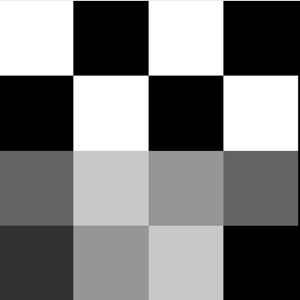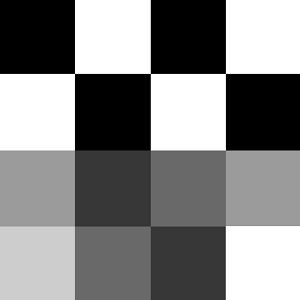# C program to invert (making negative) an image content in PGM format

Given an image in PGM format and the task is to invert the image color (making negative) content in PGM format.
Prerequisite: c-program-to-write-an-image-in-pgm-format

PGM image represents a gray scale graphic image. PGM is the abbreviation of Portable Gray Map. This image file contains one or more PGM images file.

Significance of data chunks: The data which are used to create PGM image listed below:

• P2 is the type of image that is grey image
• 4 4 is the image dimension
• 255 is the maximum grey level
• Since, the image data are stored in matrix format and each row indicates the image row and the value indicates the grey level of corresponding pixel. The maximum value (255) is used for white and minimum value (0) is used for black.

Example:

```P2
4 4
255
255 0   255 0
0   255 0   255
100 200 150 100
50  150 200 0
```

The input image looks like:How to invert the image data?
Invert the grey image means change the grey level of an image using (255 – grey lavel) i.e. if grey level of a pixel is 150 then grey level in negative image is (255 – 150) = 105. It means 255 will changes with 0 and 0 will change with 255. It is changing of proportion of black and white present in grey.
Example:

```P2
4 4
255
0   255 0   255
255 0   255 0
155 55  105 155
205  105 55 255
```

The output image looks like:`#include ` `#include ` `#include ` `void` `main() ` `{  ` `    ``int` `i, j, temp = 0; ` `    ``int` `width = 4, height = 4; ` `     `  `    ``// Suppose the 2D Array to be converted to Image  ` `    ``// is as given below  ` `    ``int` `img = { ` `        ``{255, 0, 255, 0}, ` `        ``{0, 255, 0, 255}, ` `        ``{100, 200, 150, 100}, ` `        ``{50, 150, 200, 0} ` `    ``}; ` `     `  `    ``// file pointer to store image file ` `    ``FILE` `*pgmfile, *negative_pgmfile; ` ` `  `    ``// Open an image file ` `    ``pgmfile = ``fopen``(``"img.pgm"``, ``"wb"``); ` `     `  `    ``// Open an negative image file ` `    ``negative_pgmfile = ``fopen``(``"neg_img.pgm"``, ``"wb"``); ` `     `  `    ``fprintf``(pgmfile, ``"P2 \n %d %d \n 255 \n"``, width, height); ` `    ``fprintf``(negative_pgmfile, ``"P2 \n %d %d \n 255 \n"``, width, height); ` `     `  `    ``// Create PGM image using pixel value ` `    ``for``(i = 0; i < height; i++) { ` `        ``for``(j = 0; j < width; j++) ` `            ``fprintf``(pgmfile, ``"%d "``, img[i][j]); ` `        ``fprintf``(pgmfile, ``"\n"``); ` `    ``} ` `     `  `    ``// Create negative PGM image using pixel value ` `    ``for``(i = 0; i < height; i++) { ` `        ``for``(j = 0; j < width; j++)  ` `            ``fprintf``(negative_pgmfile, ``"%d "``, (255 - img[i][j])); ` `        ``fprintf``(negative_pgmfile, ``"\n"``); ` `    ``} ` `     `  `    ``fclose``(pgmfile); ` `    ``fclose``(negative_pgmfile); ` `} `

Output:
Original ImageNegative (Invert) ImageGeeksforGeeks has prepared a complete interview preparation course with premium videos, theory, practice problems, TA support and many more features. Please refer Placement 100 for details

My Personal Notes arrow_drop_upCheck out this Author's contributed articles.

If you like GeeksforGeeks and would like to contribute, you can also write an article using contribute.geeksforgeeks.org or mail your article to contribute@geeksforgeeks.org. See your article appearing on the GeeksforGeeks main page and help other Geeks.

Please Improve this article if you find anything incorrect by clicking on the "Improve Article" button below.

Article Tags :
Practice Tags :

Be the First to upvote.

Please write to us at contribute@geeksforgeeks.org to report any issue with the above content.